# Printable Blank Number Line 1-10Updated on Sep 14, 2023By Printablee Team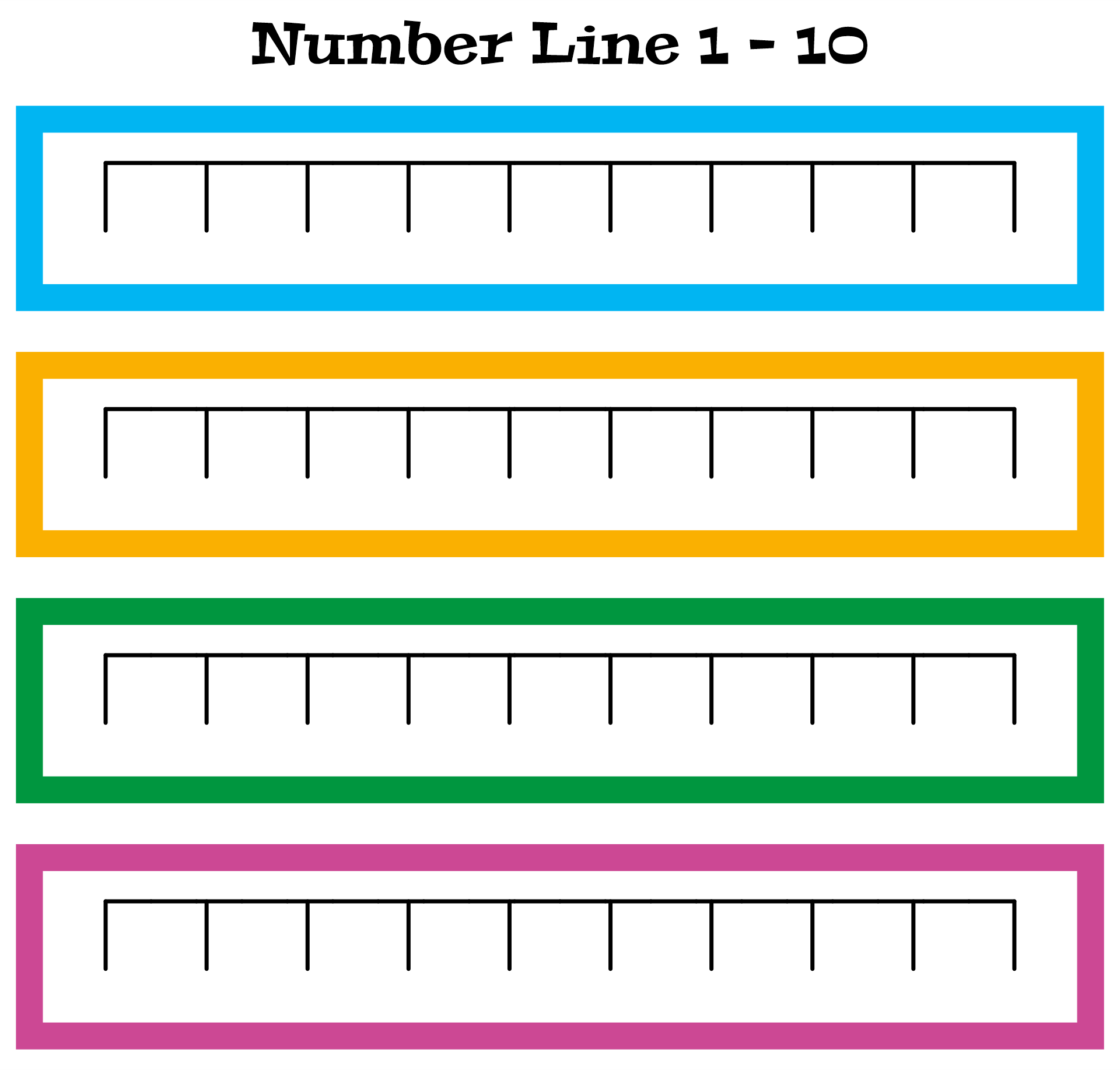## What is a Number line?

A number line is a graphical representation of numbers arranged on a straight line in ascending order. It is a mathematical tool used to represent and visualize numbers, particularly real numbers, in a linear and sequential manner. The number line is infinitely long in both positive and negative directions.

A number line typically consists of a horizontal line with a point at each end that represents a particular number. With a point designated as zero in the middle, the line is divided into equal intervals or segments. Moving further to the right from zero increases the value of the numbers to the right while moving further to the left from zero decreases the value of the numbers to the left.

Mathematical operations including addition, subtraction, multiplication, and division are frequently carried out using number lines. They aid in comprehending the placements of numbers in relation to one another, contrasting magnitudes, and spotting patterns. Number lines can be used to show distances, solve equations, analyze data on graphs, and illustrate geometric and metric principles.

## How to Apply Number Lines in Real-Life Situations?

Distances or displacements can be represented with number lines. For instance, you may use a number line to properly measure and illustrate the distance between two cities while calculating their distance along a straight route.

It is possible to comprehend temperature variations by using number lines. For instance, you may plot the morning temperature on the number line, then plot the afternoon temperature relative to the morning temperature to calculate the temperature difference from morning to afternoon. You may then see the temperature difference and measure it.

In order to keep track of financial transactions, number lines might be useful. For instance, if your bank account has a beginning value and you make deposits and withdrawals over time, you may depict these transactions on a number line to track the amount and see how it alters over time.

Effective time management can be facilitated by number lines. For instance, you may use a number line to assign time slots for various activities and make sure you utilize your time wisely if you have a set amount of time available for doing chores throughout the day.

Scaling and measuring may be done with number lines. For instance, you may use a number line to translate the measurements on a diagram to their corresponding real-world values if the design is a blueprint or a map that is scaled.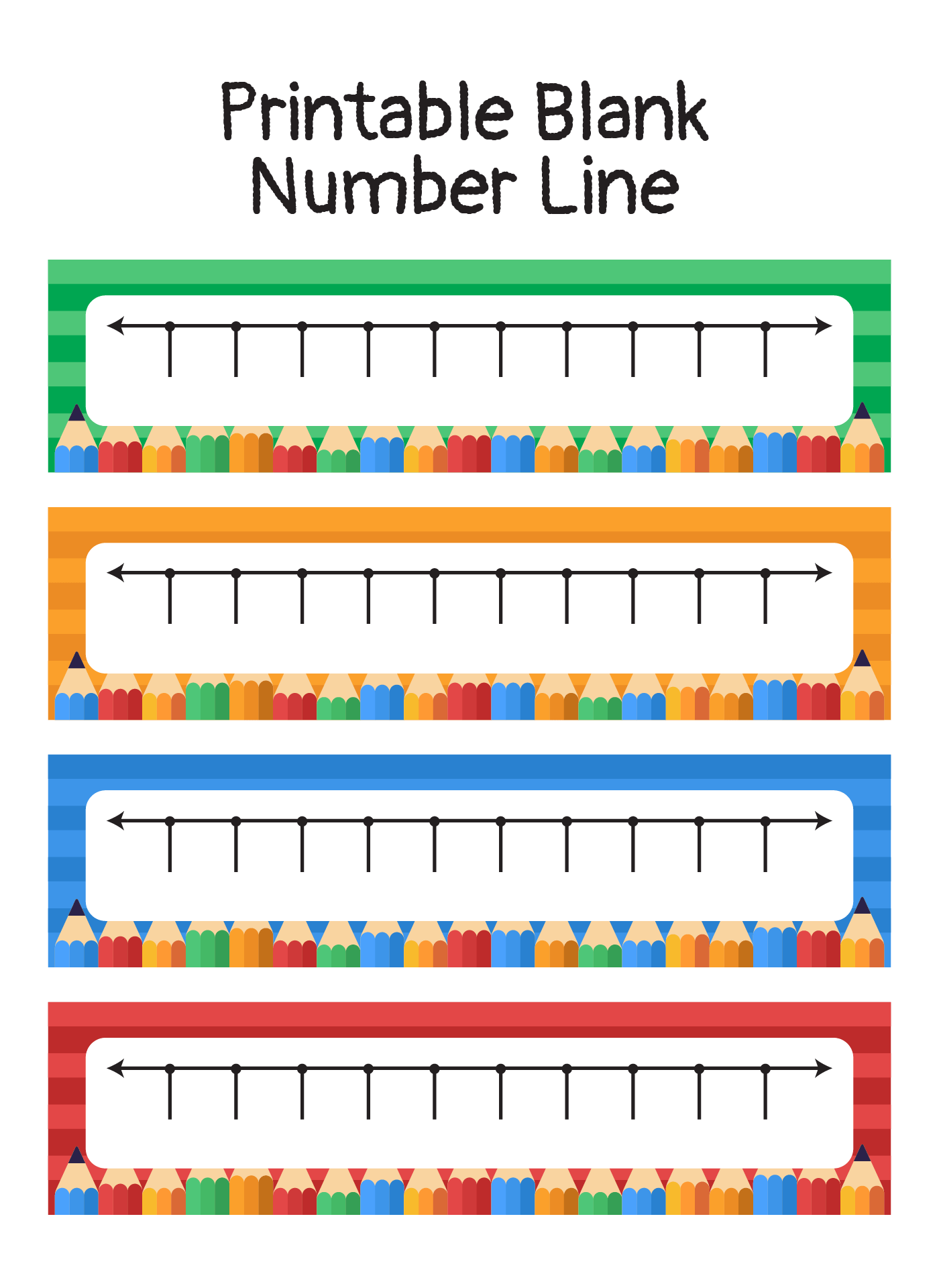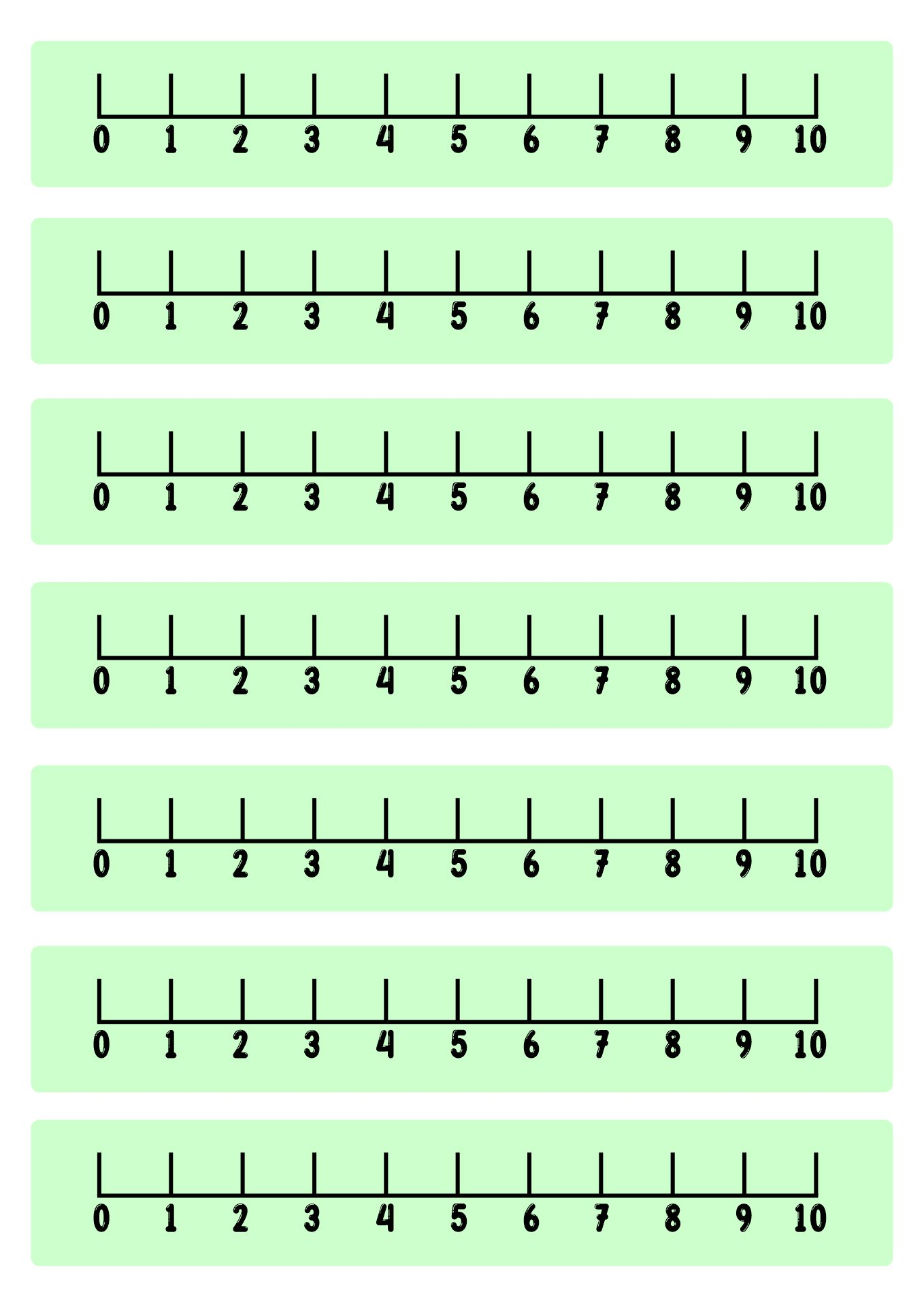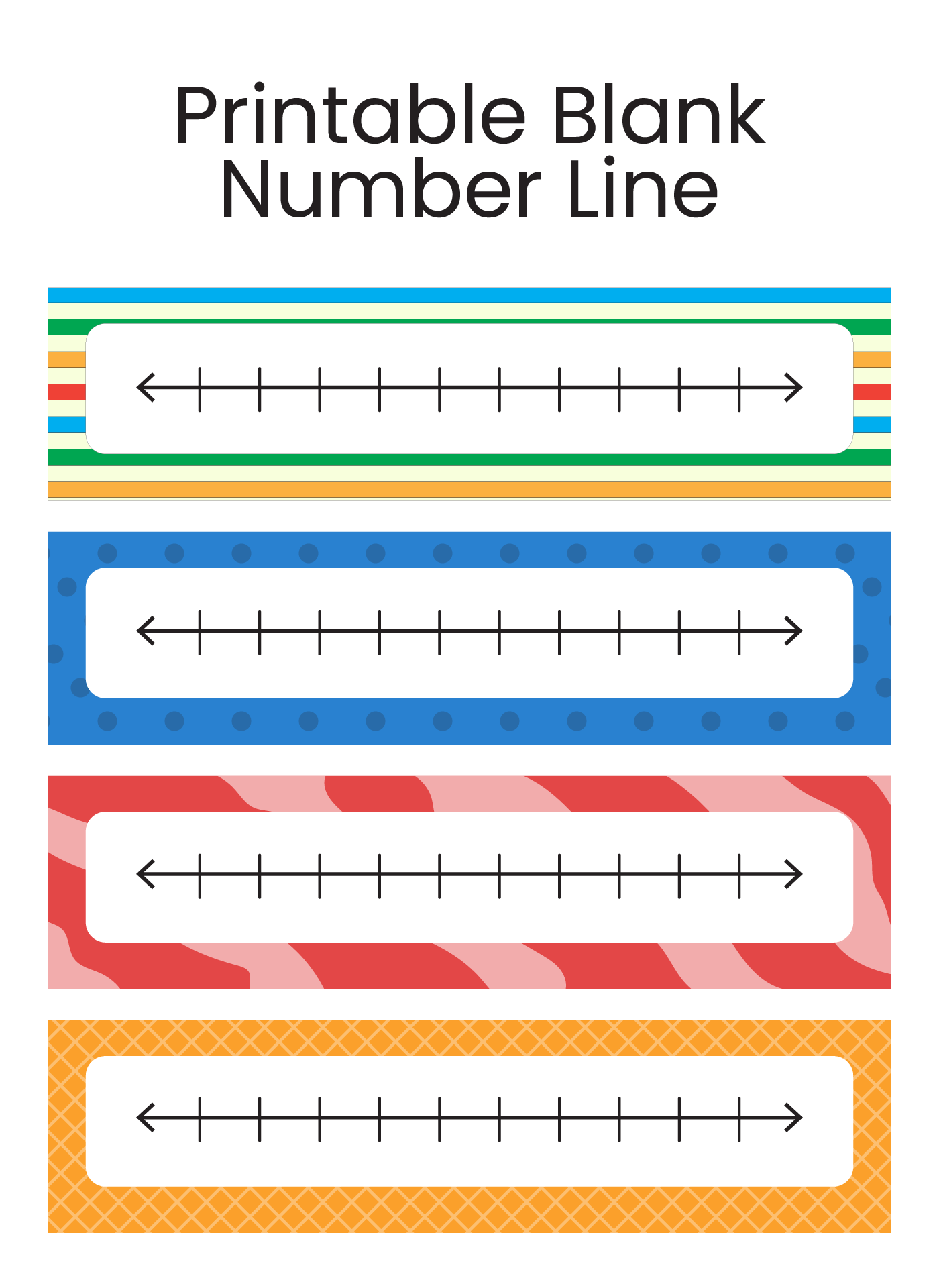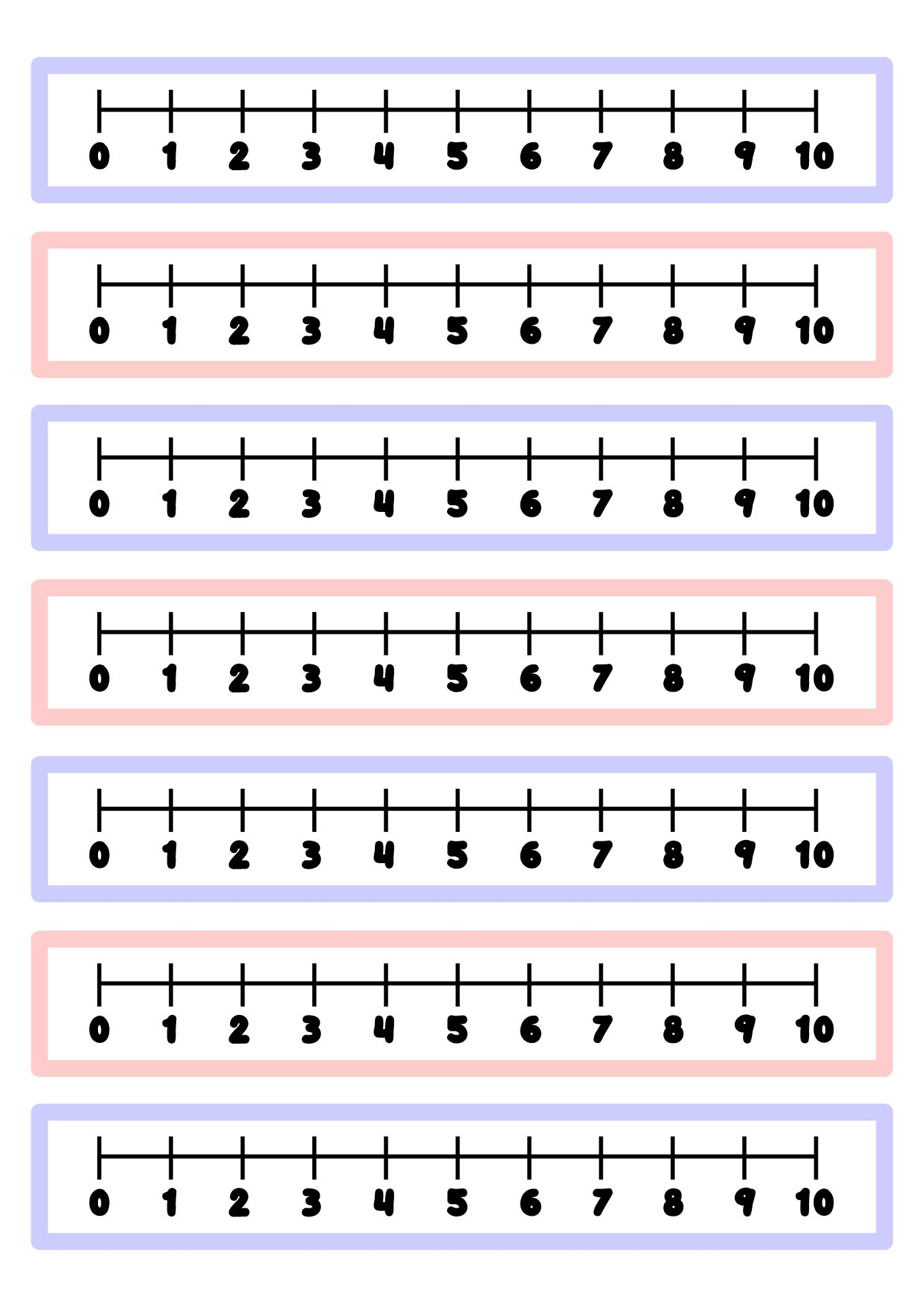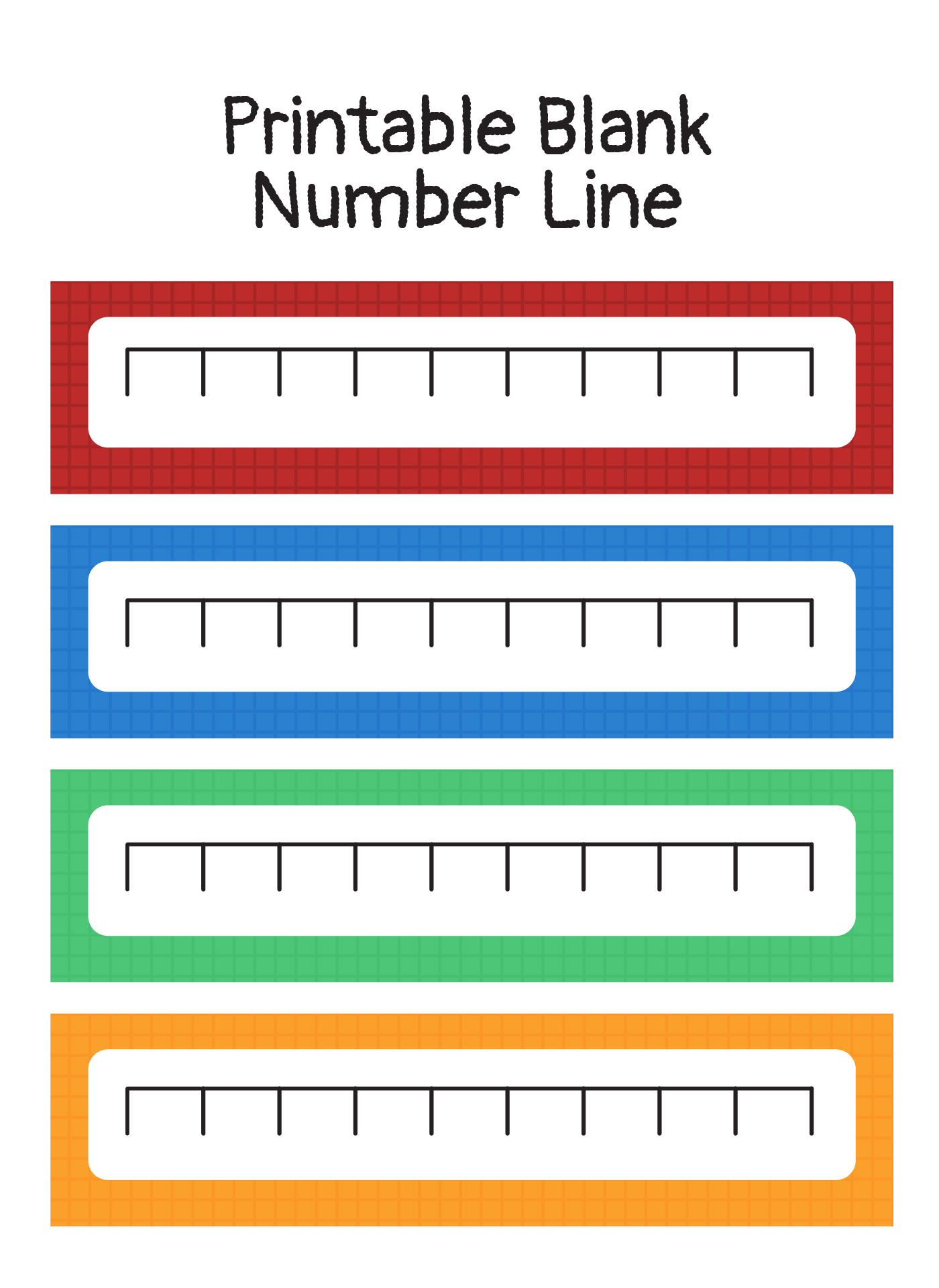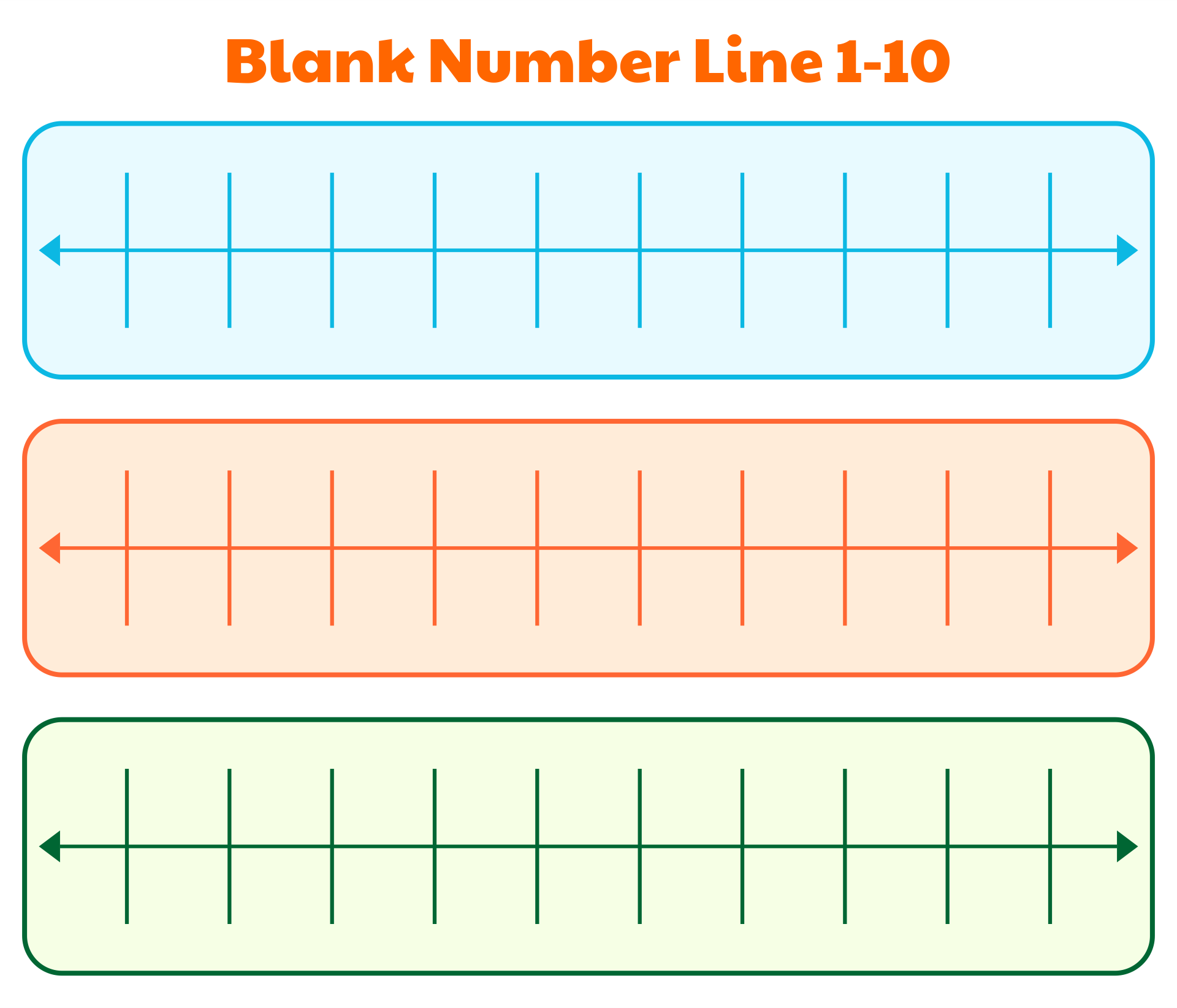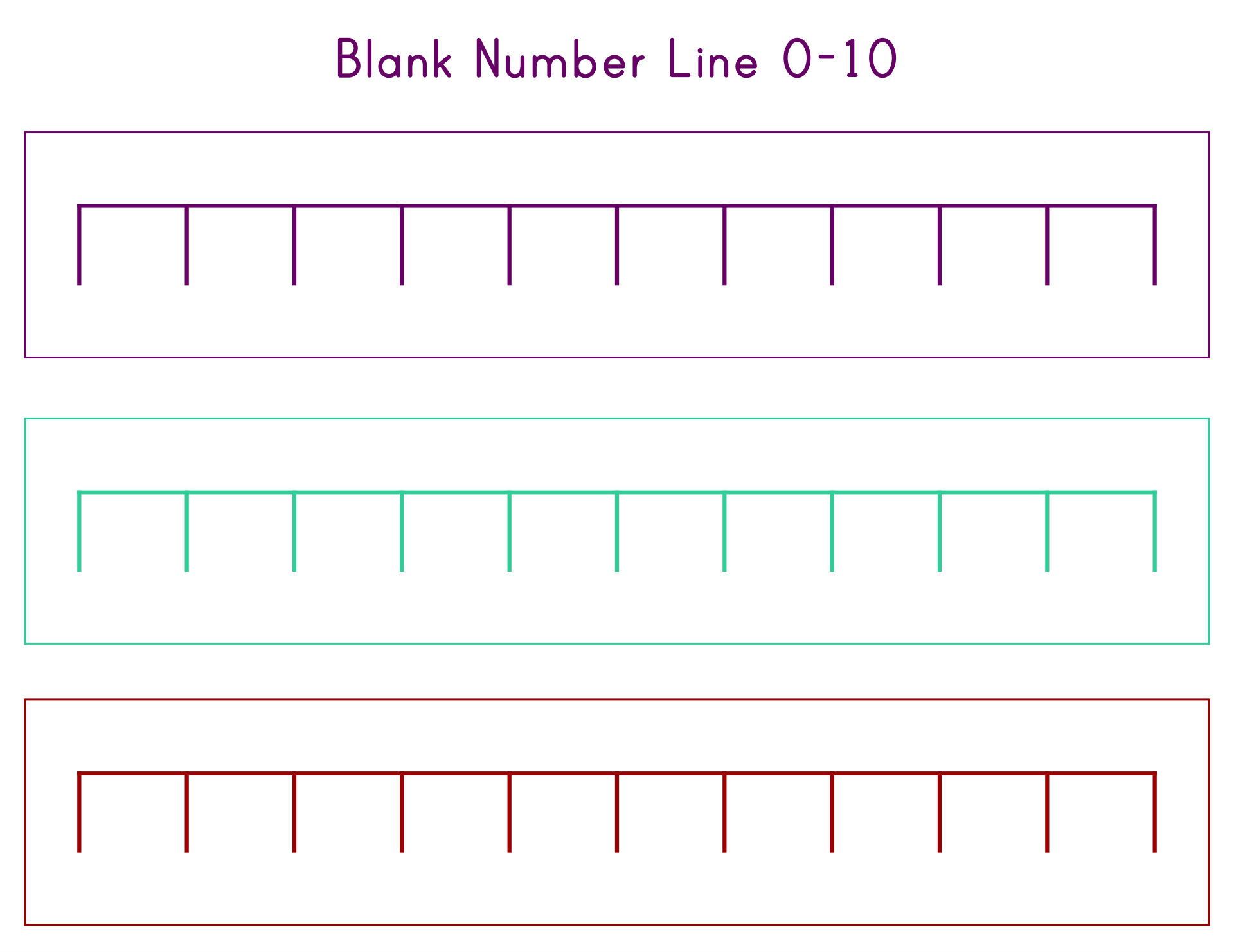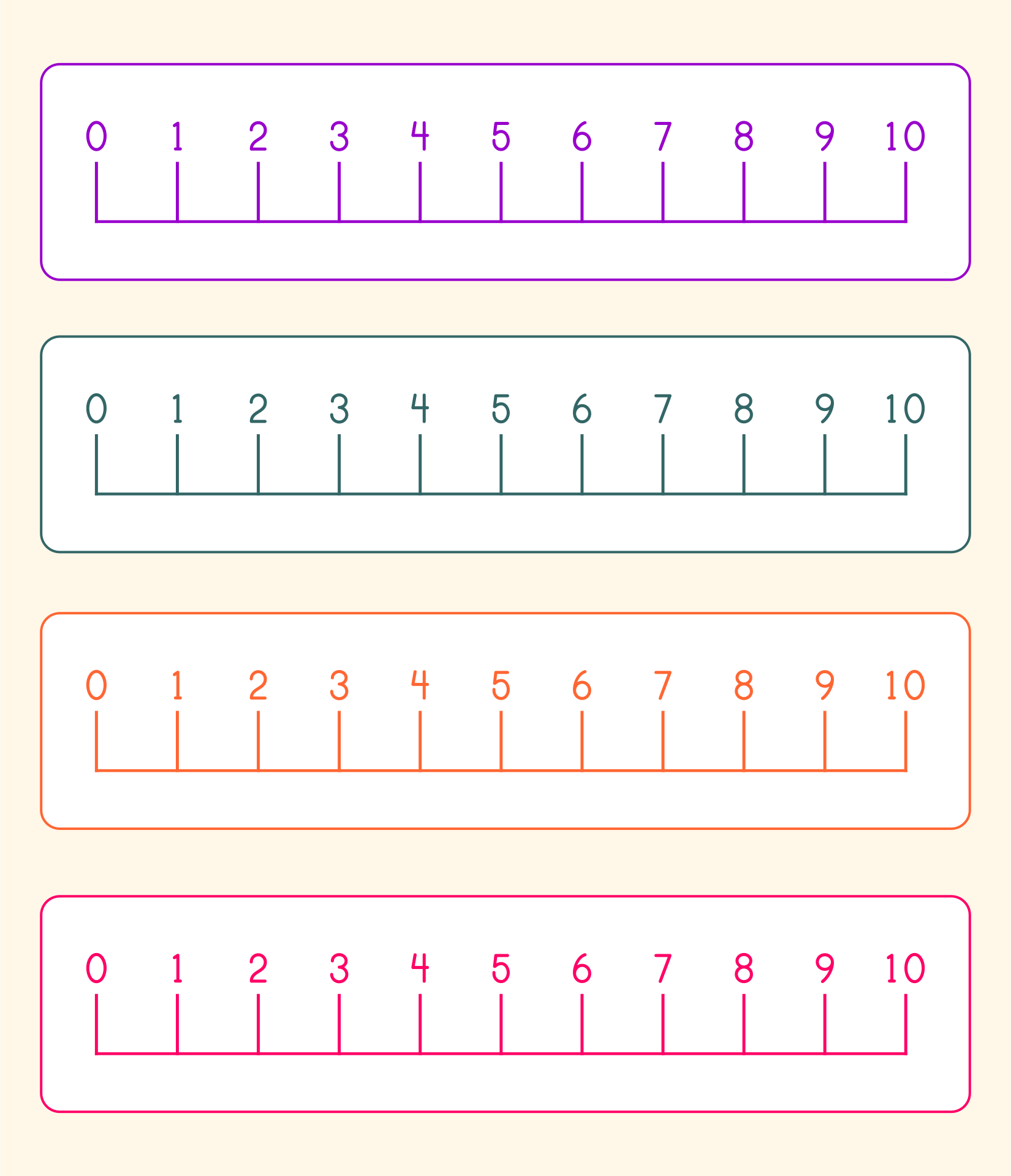## How to Teach Math Using Number Lines?

Encourage students to count the hops, evaluate the number line, and compute the final sum or difference throughout the approach. This visual representation aids in the development of practical knowledge of number connections and the effects of these operations on a number line, as well as an understanding of addition and subtraction principles.

Students can better understand comparing and organizing numbers when they use a number line to visually perceive the relationships between numbers. The number line is an excellent tool for teaching these concepts and assisting students in the development of their arithmetic skills.

### Compare and order numbers

Create a horizontal number line on the board or give each student their own number line. A suitable range for the numbers you will be comparing or ordering should be covered by the number line. Label any important values that are pertinent to the numbers being compared, such as 0, positive and negative numbers, and any other significant values, on the number line.

Pick two figures to compare. Ask the students to locate and identify these numbers on the number line. Symbol comparison should be used. Ask the students to arrange a set of numbers in ascending or descending order to learn how to order numbers.